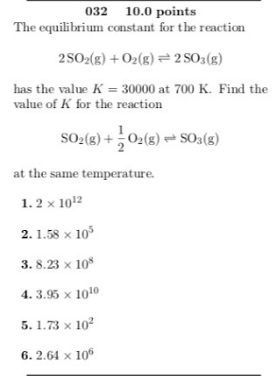# The equilibrium constant for the reaction 2 SO2(g) + O2(g) ⇌ 2 SO3(g) has he value K = 30000 at 700 K. Find the value of K for the reaction SO2(g) + 1/2O2(g) ⇌ SO3(g) at the same temperature. 1. 2 x 10^12 2. 1.58 x 10^5 3. 8.23 x 10^8 4. 3.95 x 10^10 5. 1.73 x 10^2 6. 2.64 x 10^6